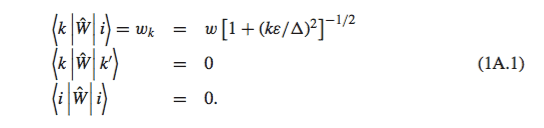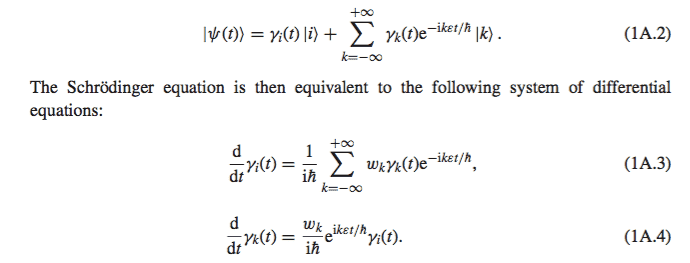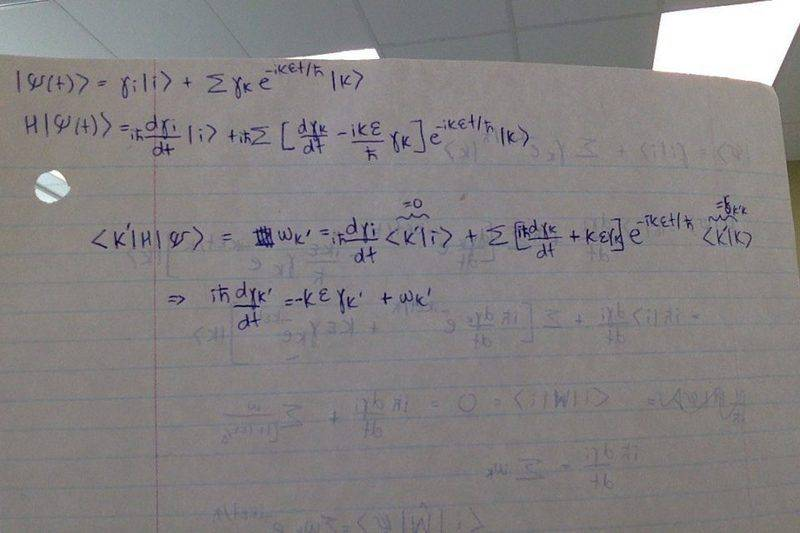# Schrödinger equation and interaction HamiltonianGiven 1A.1 and 1A.2, I have been trying to apply the Schrödinger equation to reproduce 1A.3 and 1A.4 but have been struggling a bit. I was under the assumption that by applying ##\hat{W} \rvert {\psi} \rangle= i\hbar \frac {d}{dt} \rvert{\psi} \rangle## and then taking ##\langle{k'} \lvert \hat{W} \rvert{\psi} \rangle ## and ## \langle{i}\lvert \hat{W} \rvert{\psi} \rangle## would allow me to produce 1A.3 and 1A.4. I may very well be incorrect in my methods, but did the following rough calculation and got a very different result. (In my calculation, I assumed ## \hat{W} = H ##.)Any clarification on how to reproduce 1A.3 and 1A.4 would be greatly appreciated.

blue_leaf77
Homework Helper
What are ##|i\rangle##, ##|k\rangle##, and ##w##? Moreover, how can you assume that ##H=W##? The answer of all of these questions should be available in the resource you have there.

What are ##|i\rangle##, ##|k\rangle##, and ##w##? Moreover, how can you assume that ##H=W##? The answer of all of these questions should be available in the resource you have there.

## \hat {W} ## is defined as the interaction Hamiltonian. ##|i\rangle## is the initial state the system is prepared in and ##|k\rangle## are all possible states it can evolve into where k is allowed to take all values between ## - \infty ## to ## \infty ## except ##i##.

DrClaude
Mentor
My guess is that ##\hat{H} = \hat{H}_0 + \hat{W}##, and that ##\varepsilon## has something to do with the energy in the absence of the interaction (i.e., the eigenvalue of ##\hat{H}_0##.

More details are needed. A reference would be nice.

My guess is that ##\hat{H} = \hat{H}_0 + \hat{W}##, and that ##\varepsilon## has something to do with the energy in the absence of the interaction (i.e., the eigenvalue of ##\hat{H}_0##.

More details are needed. A reference would be nice.

The initial state ## \rvert i \rangle ## has an energy equal to 0, and each state is separated in energy by a difference of ##\varepsilon##. The energy difference between ## \rvert i \rangle ## and ## \rvert k \rangle ## is k##\varepsilon##.

Sure. This is Introduction to Quantum Optics by Grynberg and this problem begins on page 34.

DrClaude
Mentor
So ##\hat{H} | k \rangle = k \varepsilon | k \rangle + \hat{W} | k \rangle##. Try that in your method.

So ##\hat{H} | k \rangle = k \varepsilon | k \rangle + \hat{W} | k \rangle##. Try that in your method.

I got it, thank you!

Just one more question, is the statement ##\langle{i} \lvert \hat{W} \rvert{k} \rangle = w_k ## necessarily true if ##\langle{k} \lvert \hat{W} \rvert{i} \rangle = w_k ## ?

If ##\hat{W} ## was an annihilation/creation operator, it seems like this definitely would not be true. Although I had to use both relations written above when reproducing 1A.3 and 1A.4. It might just be something regarding the density matrix that I missed in the text or might be implicitly assumed.

George Jones
Staff Emeritus
Gold Member
I haven't done the calculation, but is the idea to calculate ##\left<k'|H|\psi \right>## twice, once using the Schrodinger equation, and once using ##H = H_0 + W## (?) together with the facts that 1) the kets are eigenstates (as in Dr. Claude's post) of ##H_0## and 2) the relations given in the original post.

Edit: TheCanadian posted while I was writing my post. This thread seems to have a surplus of Canadians.

George Jones
Staff Emeritus
$$w_k = \frac{w}{\sqrt{1+\left(\frac{k \epsilon}{\Delta}\right)^2}}$$